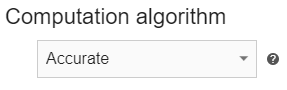# Computation algorithms

When setting up a simulation in CENOS, you will see in SIMULATION CONTROL that you are given a choice of selecting computation algorithm.

In this article we will take a look behind the computation algorithms, understand their differences and find out in which situations to use which algorithm.

## Fast​

The Fast algorithm (also weak coupling, one way coupling) calculates electromagnetic sources once and apply them to the thermal model. Reverse interaction (change of electromagnetic properties due to heating) is not taken into account.info

The fast algorithm gives good results if the time step is small compared to the heating rate.

Schematic of the calculation with Fast:## Accurate​

The accurate algorithm uses two-way coupling - electromagnetic sources are recalculated according to the temperature field until convergence is reached.info

The accurate algorithm gives very precise results with rougher time step size and is important for highly nonlinear problems.

Schematic of the calculation with Accurate:## Automatic​

Automatic algorithm switches between Fast and Accurate according to user input:

• Fast if materials have constant permeability and electrical conductivity;

• Accurate if materials have nonlinear magnetic properties (B-H model and/or temperature dependence) and temperature dependent electrical conductivity.